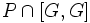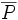# Conjugacy-closed abelian Sylow implies retract

This article gives the statement, and possibly proof, of a normal p-complement theorem: necessary and/or sufficient conditions for the existence of a Normal p-complement (?). In other words, it gives necessary and/or sufficient conditions for a given finite group to be a P-nilpotent group (?) for some prime number$p$.
View other normal p-complement theorems

## Statement

Suppose$G$ is a finite group, and$p$ is a prime dividing the order of$G$. Further, suppose$P$ is a$p$-Sylow subgroup, and$P$ is Abelian as a group, and is also conjugacy-closed: any two elements of$P$ that are conjugate in$G$ are conjugate in$P$. (Note that this effectively means that no two distinct elements of$P$ are conjugate in$G$).

Then, there exists a normal p-complement: a normal subgroup$N$ such that$NP = G$, and$N \cap P$ is trivial. In other words,$P$ is a retract of$G$.

## Related facts

### Applications

• Burnside's normal p-complement theorem: Burnside's normal p-complement theorem states that if a Sylow subgroup is central in its normalizer, it is a retract. The proof of Burnside's normal p-complement theorem relies on this fact, along with a local conjugacy-determination property in the normalizer.

## Facts used

1. Focal subgroup theorem
2. Any Abelian group is a direct product of its Sylow subgroups. In particular, a Sylow subgroup of an Abelian group is a direct factor, and has a normal complement.

## Proof

Given: A finite group$G$, a$p$-Sylow subgroup$P$ that is conjugacy-closed in$G$ and abelian.

To prove: There exists a normal$p$-complement$N$.

Proof:

1.$P \cap [G,G] = [P,P]$: By fact (1),$P \cap [G,G]$ is generated by elements of the form$x^{-1}y$ where$x,y \in P$ are conjugate in$G$. Since$P$ is conjugacy-closed in$G$, this is the same as saying that$x,y$ are conjugate in$P$, so$P \cap [G,G] = [P,P]$.
2.$P \cap [G,G]$ is trivial: This follows from the previous step, and the observation that$P$ is abelian.
3. Consider the quotient by$[G,G]$, and denote the image of$P$ as$\overline{P}$. Since$G/[G,G]$, the abelianization of$G$, is an Abelian group, and$\overline{P}$ is a Sylow subgroup, there exists a normal complement$\overline{N}$. Let$N$ be the inverse image of$\overline{N}$ under the quotient map. Clearly,$NP = G$, since it contains$[G,G]$ and its image is the whole of$G/[G,G]$. Further,$N \cap P$ is trivial, because$P$ does not intersect$[G,G]$, and the images$\overline{P}$ and$\overline{N}$ intersect trivially. Thus,$N$ is the required normal complement.